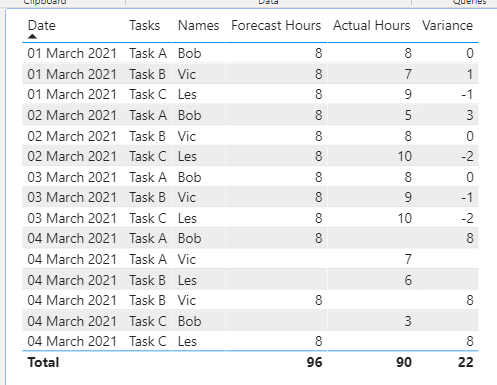cancel
Showing results for
Did you mean:Frequent Visitor

## Calculate difference between columns in two tables related by dates

Hi -

I am looking for some help trying to calculate the value of the difference between two columns that are related via a number of tables.

I have a table containing a schedule of work and another table showing what actually happened. The planned table is below:

The actual table is below:

Both tables have a number of one to many related fields for date, task, assignee as well as hours forecast/booked. The relationship table is shown below:When I try to make a calculated column on the forecast table, looking to perform Forecast Hours - Actual Hours, I cannot reference the Actual Hours column or use the RELATED function.

Please can you recommend how to approach this?

Thanks

Neil

1 ACCEPTED SOLUTIONCommunity Support

Hi @NP2020

You can create a Measure.

``````Variance =

IF (

HASONEFILTER ( Staff[Names] ),

SELECTEDVALUE ( Forecast[Hours] ) - SELECTEDVALUE ( Actual[Hours] ),

SUM ( Forecast[Hours] ) - SUM ( Actual[Hours] )

)``````

The result looks like this:For more details, you can refer the attached pbix file.

Best Regards

CaiyunZheng

Is that the answer you're looking for? If this post helps, then please consider Accept it as the solution to help the other members find it more quickly.

5 REPLIES 5Community Support

Hi @NP2020

You can create a Measure.

``````Variance =

IF (

HASONEFILTER ( Staff[Names] ),

SELECTEDVALUE ( Forecast[Hours] ) - SELECTEDVALUE ( Actual[Hours] ),

SUM ( Forecast[Hours] ) - SUM ( Actual[Hours] )

)``````

The result looks like this:For more details, you can refer the attached pbix file.

Best Regards

CaiyunZheng

Is that the answer you're looking for? If this post helps, then please consider Accept it as the solution to help the other members find it more quickly.Anonymous
Not applicable

you just don't know how you helped me a lot with this formula! Thank you so much!Frequent Visitor

Many thanks @v-cazheng-msft

Apologies for not responding sooner, I have been away.

Thanks again,

NeilSuper User

@NP2020 , You can create a new column in forecast like

Ideally with common dimensions you should be able to create a measure and use

Diff =

Sum(Forecast[Hour]) - sum(Actual[Hour])Frequent Visitor

Many thanks @amitchandak,

I have been able to implement this, although I notice that if someone books hours to a task that they were not forecast to do (highlighted below), the variance does not calculate. Is there any way to fix this?Thanks,

Neil